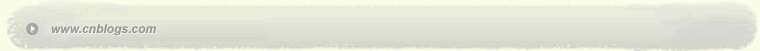茶香满园 灵感源源不断

 < 2022年6月 >
2930311234
567891011
12131415161718
19202122232425
262728293012
3456789

• 随笔 - 3
• 文章 - 7
• 评论 - 0
• 引用 - 0

# my friend

•

### 评论排行榜

1.最长公共子序列的结构

2.子问题的递归结构

3.计算最优值

Procedure LCS_LENGTH(X,Y);begin  m:=length[X];  n:=length[Y];  for i:=1 to m do c[i,j]:=0;  for j:=1 to n do c[0,j]:=0;  for i:=1 to m do    for j:=1 to n do      if x[i]=y[j] then        begin          c[i,j]:=c[i-1,j-1]+1;          b[i,j]:="↖";        end      else if c[i-1,j]≥c[i,j-1] then        begin          c[i,j]:=c[i-1,j];          b[i,j]:="↑";        end      else        begin          c[i,j]:=c[i,j-1];          b[i,j]:="←"        end;  return(c,b);end;

4.构造最长公共子序列

Procedure LCS(b,X,i,j);begin  if i=0 or j=0 then return;  if b[i,j]="↖" then    begin      LCS(b,X,i-1,j-1);      print(x[i]); {打印x[i]}    end  else if b[i,j]="↑" then LCS(b,X,i-1,j)
else LCS(b,X,i,j-1);end;

j  0  1  2  3  4  5  6
i   yj  B  D  C  A  B  A
┌ ─ ─ ─ ─ ─ ─ ─ ─ ─ ─ ─ ─ ─ ┐
0 xi │   0  0  0  0  0  0 │
│   ↑  ↑  ↑ ↖    ↖  │
1 A │ 0  0  0  0  1 ← 1  1 │
│  ↖       ↑ ↖    │
2 B │ 0  1 ← 1 ← 1  1  2 ← 2 │
│   ↑  ↑ ↖     ↑  ↑ │
3 C │ 0  1  1  2 ← 2  2  2 │
│  ↖   ↑  ↑  ↑ ↖    │
4 B │ 0  1  1  2  2  3 ← 3 │
│   ↑ ↖   ↑  ↑  ↑  ↑ │
5 D │ 0  1  2  2  2  3  3 │
│   ↑  ↑  ↑ ↖   ↑ ↖  │
6 A │ 0  1  2  2  3  3  4 │
│  ↖   ↑  ↑  ↑ ↖   ↑ │
7 B │ 0  1  2  2  3  4  5 │
└ ─ ─ ─ ─ ─ ─ ─ ─ ─ ─ ─ ─ ─ ┘

5.算法的改进

posted on 2006-05-26 09:41 小茶 阅读(520) 评论(0)  编辑 收藏 引用 所属分类: 算法Copyright © 小茶 Powered by: 博客园 模板提供：沪江博客